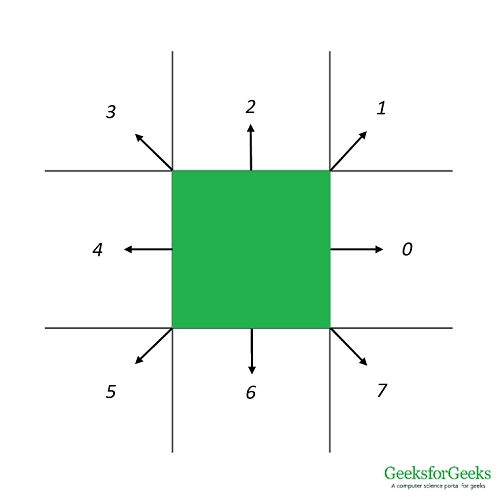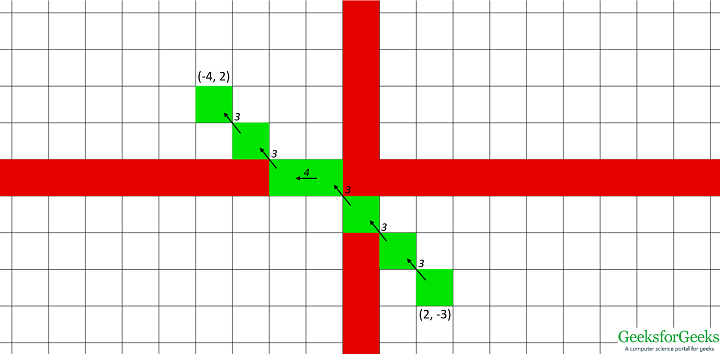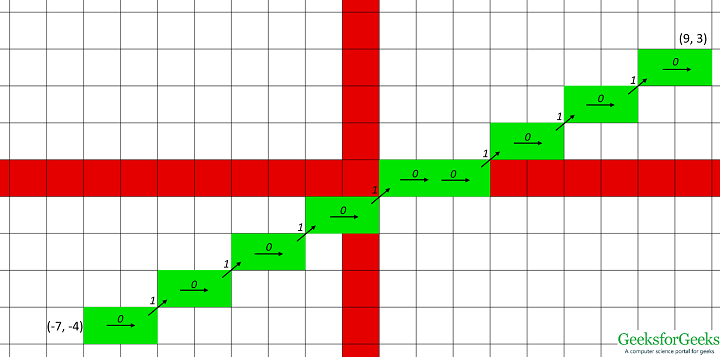# Chain Code for 2D Line

Chain code is a lossless compression technique used for representing an object in images. The co-ordinates of any continuous boundary of an object can be represented as a string of numbers where each number represents a particular direction in which the next point on the connected line is present. One point is taken as the reference/starting point and on plotting the points generated from the chain, the original figure can be re-drawn.

This article describes how to generate a 8-neighbourhood chain code of a 2-D straight line. In a rectangular grid, a point can have at most 8 surrounding points as shown below. The next point on the line has to be one of these 8 surrounding points. Each direction is assigned a code. Using this code we can find out which of the surrounding point should be plotted next.The chain codes could be generated by using conditional statements for each direction but it becomes very tedious to describe for systems having large number of directions(3-D grids can have up to 26 directions). Instead we use a hash function. The difference in X() and Y() co-ordinates of two successive points are calculated and hashed to generate the key for the chain code between the two points.

Chain code list:Hash function:Hash table:-1 0 5 0
1 1 8 1
0 1 7 2
-1 1 6 3
-1 0 3 4
-1 -1 0 5
0 -1 1 6
1 -1 2 7

The function does not generate the value 4 so a dummy value is stored there.

Examples:

```Input : (2, -3), (-4, 2)
Output : Chain code for the straight line from (2, -3) to (-4, 2) is 333433Input : (-7, -4), (9, 3)
Output : Chain code for the straight line from (-7, -4) to (9, 3) is 0101010100101010```

## Python3

 `# Python3 code for generating 8-neighbourhood chain ` `# code for a 2-D line ` ` `  `codeList ``=` `[``5``, ``6``, ``7``, ``4``, ``-``1``, ``0``, ``3``, ``2``, ``1``] ` ` `  `# This function generates the chaincode  ` `# for transition between two neighbour points ` `def` `getChainCode(x1, y1, x2, y2): ` `    ``dx ``=` `x2 ``-` `x1 ` `    ``dy ``=` `y2 ``-` `y1 ` `    ``hashKey ``=` `3` `*` `dy ``+` `dx ``+` `4` `    ``return` `codeList[hashKey] ` ` `  `'''This function generates the list of  ` `chaincodes for given list of points'''` `def` `generateChainCode(ListOfPoints): ` `    ``chainCode ``=` `[] ` `    ``for` `i ``in` `range``(``len``(ListOfPoints) ``-` `1``): ` `        ``a ``=` `ListOfPoints[i] ` `        ``b ``=` `ListOfPoints[i ``+` `1``] ` `        ``chainCode.append(getChainCode(a[``0``], a[``1``], b[``0``], b[``1``])) ` `    ``return` `chainCode ` ` `  ` `  `'''This function generates the list of points for  ` `a staright line using Bresenham's Algorithm'''` `def` `Bresenham2D(x1, y1, x2, y2): ` `    ``ListOfPoints ``=` `[] ` `    ``ListOfPoints.append([x1, y1]) ` `    ``xdif ``=` `x2 ``-` `x1 ` `    ``ydif ``=` `y2 ``-` `y1 ` `    ``dx ``=` `abs``(xdif) ` `    ``dy ``=` `abs``(ydif) ` `    ``if``(xdif > ``0``): ` `        ``xs ``=` `1` `    ``else``: ` `        ``xs ``=` `-``1` `    ``if` `(ydif > ``0``): ` `        ``ys ``=` `1` `    ``else``: ` `        ``ys ``=` `-``1` `    ``if` `(dx > dy): ` ` `  `        ``# Driving axis is the X-axis ` `        ``p ``=` `2` `*` `dy ``-` `dx ` `        ``while` `(x1 !``=` `x2): ` `            ``x1 ``+``=` `xs ` `            ``if` `(p >``=` `0``): ` `                ``y1 ``+``=` `ys ` `                ``p ``-``=` `2` `*` `dx ` `            ``p ``+``=` `2` `*` `dy ` `            ``ListOfPoints.append([x1, y1]) ` `    ``else``: ` ` `  `        ``# Driving axis is the Y-axis ` `        ``p ``=` `2` `*` `dx``-``dy ` `        ``while``(y1 !``=` `y2): ` `            ``y1 ``+``=` `ys ` `            ``if` `(p >``=` `0``): ` `                ``x1 ``+``=` `xs ` `                ``p ``-``=` `2` `*` `dy ` `            ``p ``+``=` `2` `*` `dx ` `            ``ListOfPoints.append([x1, y1]) ` `    ``return` `ListOfPoints ` ` `  `def` `DriverFunction(): ` `    ``(x1, y1) ``=` `(``-``9``, ``-``3``) ` `    ``(x2, y2) ``=` `(``10``, ``1``) ` `    ``ListOfPoints ``=` `Bresenham2D(x1, y1, x2, y2) ` `    ``chainCode ``=` `generateChainCode(ListOfPoints) ` `    ``chainCodeString ``=` `"".join(``str``(e) ``for` `e ``in` `chainCode) ` `    ``print` `(``'Chain code for the straight line from'``, (x1, y1),  ` `            ``'to'``, (x2, y2), ``'is'``, chainCodeString) ` ` `  `DriverFunction() `

Output:

```Chain code for the straight line from (-9, -3) to (10, 1) is 0010000100010000100
```

Don’t stop now and take your learning to the next level. Learn all the important concepts of Data Structures and Algorithms with the help of the most trusted course: DSA Self Paced. Become industry ready at a student-friendly price.

My Personal Notes arrow_drop_upCheck out this Author's contributed articles.

If you like GeeksforGeeks and would like to contribute, you can also write an article using contribute.geeksforgeeks.org or mail your article to contribute@geeksforgeeks.org. See your article appearing on the GeeksforGeeks main page and help other Geeks.

Please Improve this article if you find anything incorrect by clicking on the "Improve Article" button below.

Article Tags :
Practice Tags :

Be the First to upvote.

Please write to us at contribute@geeksforgeeks.org to report any issue with the above content.